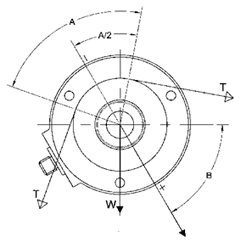# Load Cell Sizing: Slim CellMWF = T x K x sin (A/2) ± (W/2) x sin (B)* *Notes: The MWF calculation defines the force on each individual load cell. If Angle B is below horizontal use + (plus) in the calculation. If Angle B is above horizontal use - (minus) in the calculation.